# Sum and product

The sum of the real numbers x and y is 12. Their difference is 8. What is the value of xy?

w =  20

### Step-by-step explanation: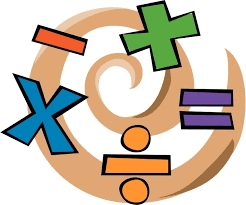Did you find an error or inaccuracy? Feel free to write us. Thank you!Tips to related online calculators
Do you have a system of equations and looking for calculator system of linear equations?

## Related math problems and questions:

• The sum 15The sum of the real numbers x and y is 24. Their difference is 12. What is the value of xy?
• The sum 9The sum of two integers is 4. Their difference is 8. What are this two integers?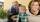Grandson with grandpa, they counted how many years have together. Their product is 365. How many years is the sum of their years?
• The difference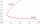The difference of two numbers is 1375. If their exact quotient is 12. Find the two numbers
• The difference 2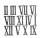The difference between the two numbers is 25. The smaller number is 1/6th of the larger number. What is the value of the smaller number?
• The sum 16The sum of A and B is 36. The difference of A & B is 8. find the larger number of A.
• Product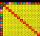The sum and the product of three integers are 6. Write largest of them.
• Sum and ratioThe sum and ratio of two numbers is equal to 10. Which numbers are they?
• Find twoFind two consecutive natural numbers whose product is 1 larger than their sum. Searched numbers expressed by a fraction whose numerator is the difference between these numbers and the denominator is their sum.
• Product of the sum and differenceCalculate the product of the sum and difference of numbers -7 and -2.
• Value 4If 5/18=425, what is the value 13/18?
• Three numbersFind three numbers so that the second number is 4 times greater than the first and the third is lower by 5 than the second number. Their sum is 67.
• ABS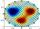What is the value of ? ?
• Complicated sum minus productWhat must be subtracted from the sum of 3/8 and 5/16 to get difference equal to the product of 5/8 and 3/16?
• Three men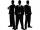Alex is half younger than Jan, which is one-third younger than George. The sum of their ages is 48. How are these three men old?
• Sum or productWhat is the probability that two dice fall will have the sum 7 or product 12?
• Two numbersThe sum of two numbers is 1. Identify this two numbers if you know that the half of first is equal to the third of second number.